### Home > A2C > Chapter 13 > Lesson 13.1.3 > Problem13-56

13-56.
1. For each of the following problems, find all of the solutions without using a calculator. Use a graph or unit circle to support you answers. Then predict the solution that your calculator will give and use your calculator to check your prediction. Homework Help ✎

1.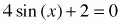2.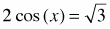3.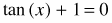4.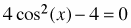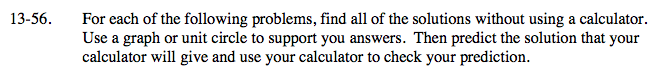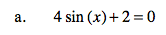$\text{sin}(x)=-\frac{1}{2}$

$x=\frac{7\pi}{6}+2\pi n,\,\,x=\frac{11\pi}{6}+2\pi n$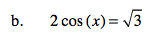See part (a).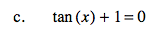See part (a).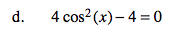cos2(x) = 1

x = πn